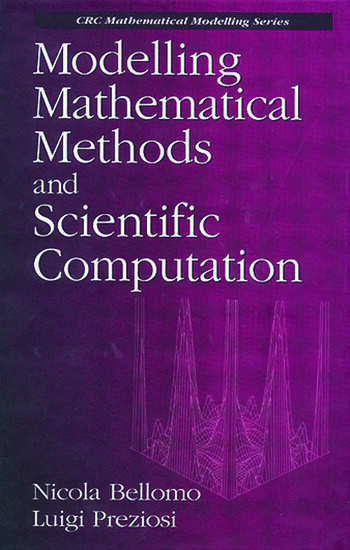Modelling Mathematical Methods and Scientific Computation

1st Edition

Nicola Bellomo, Luigi Preziosi

CRC Press
Published December 22, 1994
Reference - 512 Pages
ISBN 9780849383311 - CAT# 8331
Series: Mathematical Modeling

For Instructors Request Inspection Copy

USD\$230.00

FREE Standard Shipping!

Preview

Summary

Addressed to engineers, scientists, and applied mathematicians, this book explores the fundamental aspects of mathematical modelling in applied sciences and related mathematical and computational methods. After providing the general framework needed for mathematical modelling-definitions, classifications, general modelling procedures, and validation methods-the authors deal with the analysis of discrete models. This includes modelling methods and related mathematical methods. The analysis of models is defined in terms of ordinary differential equations. The analysis of continuous models, particularly models defined in terms of partial differential equations, follows. The authors then examine inverse type problems and stochastic modelling. Three appendices provide a concise guide to functional analysis, approximation theory, and probability, and a diskette included with the book includes ten scientific programs to introduce the reader to scientific computation at a practical level.

Instructors

We provide complimentary e-inspection copies of primary textbooks to instructors considering our books for course adoption.